Test: Basic Concepts Of Differential And Integral Calculus- 1

# Test: Basic Concepts Of Differential And Integral Calculus- 1

Test Description

## 40 Questions MCQ Test Quantitative Aptitude for CA CPT | Test: Basic Concepts Of Differential And Integral Calculus- 1

Test: Basic Concepts Of Differential And Integral Calculus- 1 for CA Foundation 2022 is part of Quantitative Aptitude for CA CPT preparation. The Test: Basic Concepts Of Differential And Integral Calculus- 1 questions and answers have been prepared according to the CA Foundation exam syllabus.The Test: Basic Concepts Of Differential And Integral Calculus- 1 MCQs are made for CA Foundation 2022 Exam. Find important definitions, questions, notes, meanings, examples, exercises, MCQs and online tests for Test: Basic Concepts Of Differential And Integral Calculus- 1 below.
Solutions of Test: Basic Concepts Of Differential And Integral Calculus- 1 questions in English are available as part of our Quantitative Aptitude for CA CPT for CA Foundation & Test: Basic Concepts Of Differential And Integral Calculus- 1 solutions in Hindi for Quantitative Aptitude for CA CPT course. Download more important topics, notes, lectures and mock test series for CA Foundation Exam by signing up for free. Attempt Test: Basic Concepts Of Differential And Integral Calculus- 1 | 40 questions in 40 minutes | Mock test for CA Foundation preparation | Free important questions MCQ to study Quantitative Aptitude for CA CPT for CA Foundation Exam | Download free PDF with solutions
 1 Crore+ students have signed up on EduRev. Have you?
Test: Basic Concepts Of Differential And Integral Calculus- 1 - Question 1

### Choose the most appropriate option (a) (b) (c) or (d) The gradient of the curve y = 2x3 –3x2 – 12x +8 at x = 0 is

Detailed Solution for Test: Basic Concepts Of Differential And Integral Calculus- 1 - Question 1

Solution :- 2x^3 - 3x^2 -12x + 8 = 0

dy/dx = 6x^2 - 6x -12 = 0

(At x=0) = 6(0)^2 - 6(0) - 12

= -12

Test: Basic Concepts Of Differential And Integral Calculus- 1 - Question 2

### The gradient of the curve y = 2x3 –5x2 – 3x at x = 0 is

Test: Basic Concepts Of Differential And Integral Calculus- 1 - Question 3

### The derivative of y =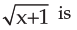Test: Basic Concepts Of Differential And Integral Calculus- 1 - Question 4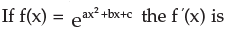Test: Basic Concepts Of Differential And Integral Calculus- 1 - Question 5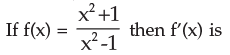Test: Basic Concepts Of Differential And Integral Calculus- 1 - Question 6

If y = x (x –1) (x – 2) then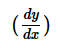is

Test: Basic Concepts Of Differential And Integral Calculus- 1 - Question 7

The gradient of the curve y – xy + 2px + 3qy = 0 at the point (3, 2 ) is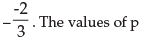and q are

Detailed Solution for Test: Basic Concepts Of Differential And Integral Calculus- 1 - Question 7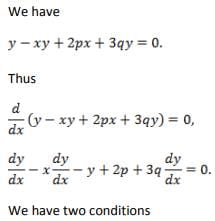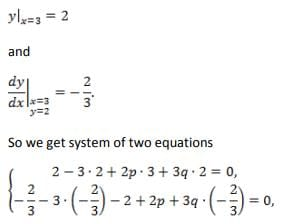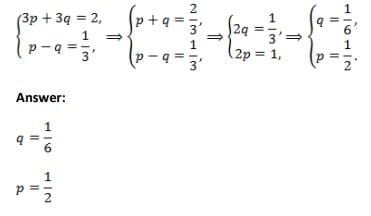Test: Basic Concepts Of Differential And Integral Calculus- 1 - Question 8

The curve y2 = ux3 + v passes through the point P(2, 3) and= 4 at P. The values of u and v are

Detailed Solution for Test: Basic Concepts Of Differential And Integral Calculus- 1 - Question 8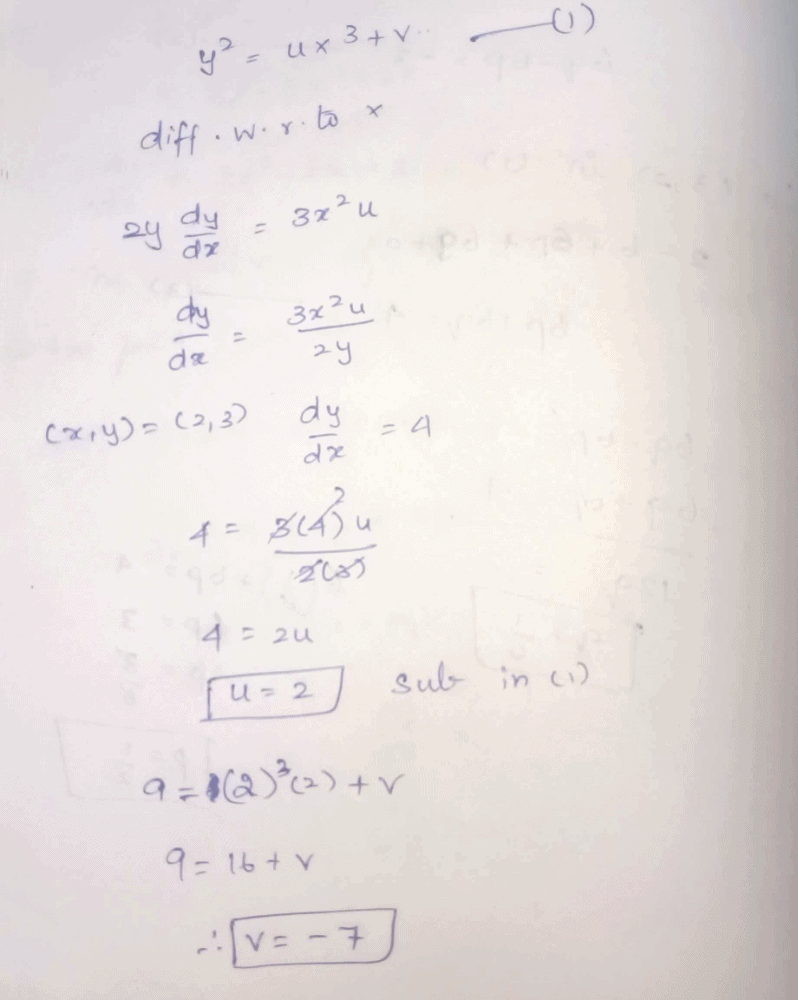Test: Basic Concepts Of Differential And Integral Calculus- 1 - Question 9

The gradient of the curve y + px +qy = 0 at (1, 1) is 1/2. The values of p and q are

Detailed Solution for Test: Basic Concepts Of Differential And Integral Calculus- 1 - Question 9

y+px+qy=0
y+qy=-px
(1+q)y=-px
y=-[p/(1+q)]x
This is a linear function, so has constant gradient at all points on the curve. Hence
-p/(1+q)=1/2
2p=-(1+q)
But there is an issue: you have stated that the curve's gradient is 1/2 at the point (1,1) but the curve does not cross through this point! Regardless of our choices for p and q satisfying the expressions above this paragraph, the equation of the curve will always simplify to y=0.5x, which crosses through the origin (0,0), as well as (1,0.5) and (2,1) - but not (-1,1).
For your curve to pass through (-1,1), we would need to add a constant term, like so:
y+px+qy=1/2

Test: Basic Concepts Of Differential And Integral Calculus- 1 - Question 10

If xy = 1 then y2 + dy/dx is equal to

Test: Basic Concepts Of Differential And Integral Calculus- 1 - Question 11

The derivative of the function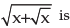Test: Basic Concepts Of Differential And Integral Calculus- 1 - Question 12

Given e-xy –4xy = 0,can be proved to be

Test: Basic Concepts Of Differential And Integral Calculus- 1 - Question 13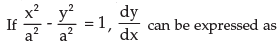Detailed Solution for Test: Basic Concepts Of Differential And Integral Calculus- 1 - Question 13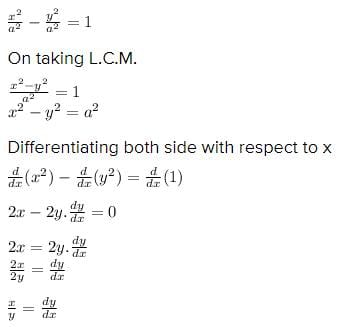Test: Basic Concepts Of Differential And Integral Calculus- 1 - Question 14

If log (x / y) = x + y,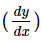may be found to be

Test: Basic Concepts Of Differential And Integral Calculus- 1 - Question 15

If f(x, y) = x3 + y3 – 3axy = 0,can be found out as

Test: Basic Concepts Of Differential And Integral Calculus- 1 - Question 16

Given x = at2, y = 2at;is calculated as

Test: Basic Concepts Of Differential And Integral Calculus- 1 - Question 17

Given x = 2t + 5, y = t2 – 2;is calculated as

Test: Basic Concepts Of Differential And Integral Calculus- 1 - Question 18

If y =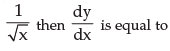Test: Basic Concepts Of Differential And Integral Calculus- 1 - Question 19

If x = 3t2 –1, y = t3 –t, thenis equal to

Test: Basic Concepts Of Differential And Integral Calculus- 1 - Question 20

The slope of the tangent to the curve y =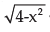at the point, where the ordinate and the abscissa are equal, is

Detailed Solution for Test: Basic Concepts Of Differential And Integral Calculus- 1 - Question 20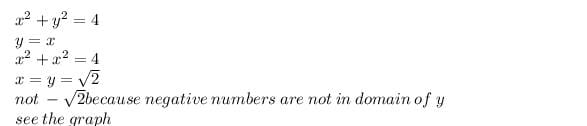so the point is (√2,√2)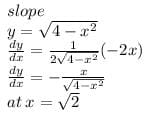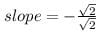= -1

Test: Basic Concepts Of Differential And Integral Calculus- 1 - Question 21

The slope of the tangent to the curve y = x2 –x at the point, where the line y = 2 cuts the curve in the Ist quadrant, is

Test: Basic Concepts Of Differential And Integral Calculus- 1 - Question 22

For the curve x2 + y2 + 2gx + 2hy = 0, the value ofat (0, 0) is

Test: Basic Concepts Of Differential And Integral Calculus- 1 - Question 23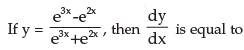Test: Basic Concepts Of Differential And Integral Calculus- 1 - Question 24

If xy.yx = M, M is constant thenis equal to

Test: Basic Concepts Of Differential And Integral Calculus- 1 - Question 25

Given x = t + t–1 and y = t – t–1 the value ofat t = 2 is

Test: Basic Concepts Of Differential And Integral Calculus- 1 - Question 26

If x3 –2x2 y2 + 5x +y –5 =0 thenat x = 1, y = 1 is equal to

Test: Basic Concepts Of Differential And Integral Calculus- 1 - Question 27

The derivative of x2 log x is

Test: Basic Concepts Of Differential And Integral Calculus- 1 - Question 28

The derivative of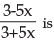Test: Basic Concepts Of Differential And Integral Calculus- 1 - Question 29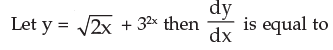Test: Basic Concepts Of Differential And Integral Calculus- 1 - Question 30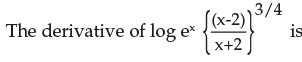Test: Basic Concepts Of Differential And Integral Calculus- 1 - Question 31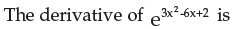Test: Basic Concepts Of Differential And Integral Calculus- 1 - Question 32

If f(x) = 3x2, then F(x) =

Test: Basic Concepts Of Differential And Integral Calculus- 1 - Question 33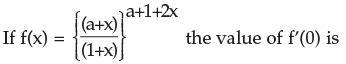Test: Basic Concepts Of Differential And Integral Calculus- 1 - Question 34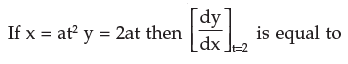Test: Basic Concepts Of Differential And Integral Calculus- 1 - Question 35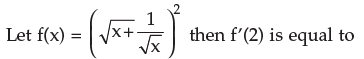Test: Basic Concepts Of Differential And Integral Calculus- 1 - Question 36

If f(x) = x2 – 6x+8 then f ’(5) – f ’(8) is equal to

Test: Basic Concepts Of Differential And Integral Calculus- 1 - Question 37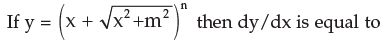Test: Basic Concepts Of Differential And Integral Calculus- 1 - Question 38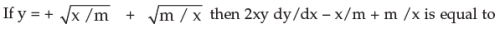Test: Basic Concepts Of Differential And Integral Calculus- 1 - Question 39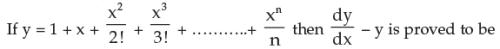Test: Basic Concepts Of Differential And Integral Calculus- 1 - Question 40

If f(x) = xk and f’(1) = 10 the value of k is

## Quantitative Aptitude for CA CPT

67 docs|68 tests
 Use Code STAYHOME200 and get INR 200 additional OFF Use Coupon Code
Information about Test: Basic Concepts Of Differential And Integral Calculus- 1 Page
In this test you can find the Exam questions for Test: Basic Concepts Of Differential And Integral Calculus- 1 solved & explained in the simplest way possible. Besides giving Questions and answers for Test: Basic Concepts Of Differential And Integral Calculus- 1, EduRev gives you an ample number of Online tests for practice

67 docs|68 tests